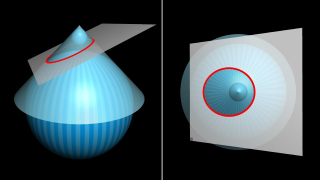# Conic section

Topic | v1 | created by janarez |Description

In mathematics, a conic section (or simply conic) is a curve obtained as the intersection of the surface of a cone with a plane. The three types of conic section are the hyperbola, the parabola, and the ellipse; the circle is a special case of the ellipse, though historically it was sometimes called a fourth type. The ancient Greek mathematicians studied conic sections, culminating around 200 BC with Apollonius of Perga's systematic work on their properties. The conic sections in the Euclidean plane have various distinguishing properties, many of which can be used as alternative definitions. One such property defines a non-circular conic to be the set of those points whose distances to some particular point, called a focus, and some particular line, called a directrix, are in a fixed ratio, called the eccentricity. The type of conic is determined by the value of the eccentricity.

##### Relations

Euclidean geometry is a mathematical system attributed to Alexandrian Greek mathematician Euclid, whi...##### Resources

10.0 rating 2.0 level 10.0 clarity 5.0 background – 1 rating

Dandelin spheres, conic sections, and a view of genius in math.